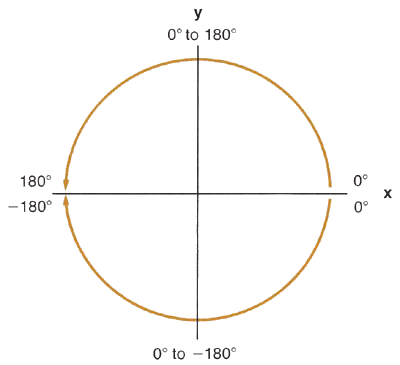Section 6: Functions93
In the HP-41C, angle θ is represented in the following manner:To convert from rectangular x, y coordinates to polar (r, θ) coordinates (magnitude and angle, respectively):
 1. Key in the the y-coordinate. 2. Press ENTER  . 3. Key in the the x-coordinate. 4. Execute R–P (rectangular to polar). Magnitude r is placed in the X-register and angle θ is placed in the Y-register. To display the θ value, press X Y .
When you execute the R–P function, the stack contents are changed...
...from this...           ...to this.

 T t

 T t

 Z z

 Z z

 Y y-coordinate
R–P
 Y angle θ

 X x-coordinate

 X magnitude r